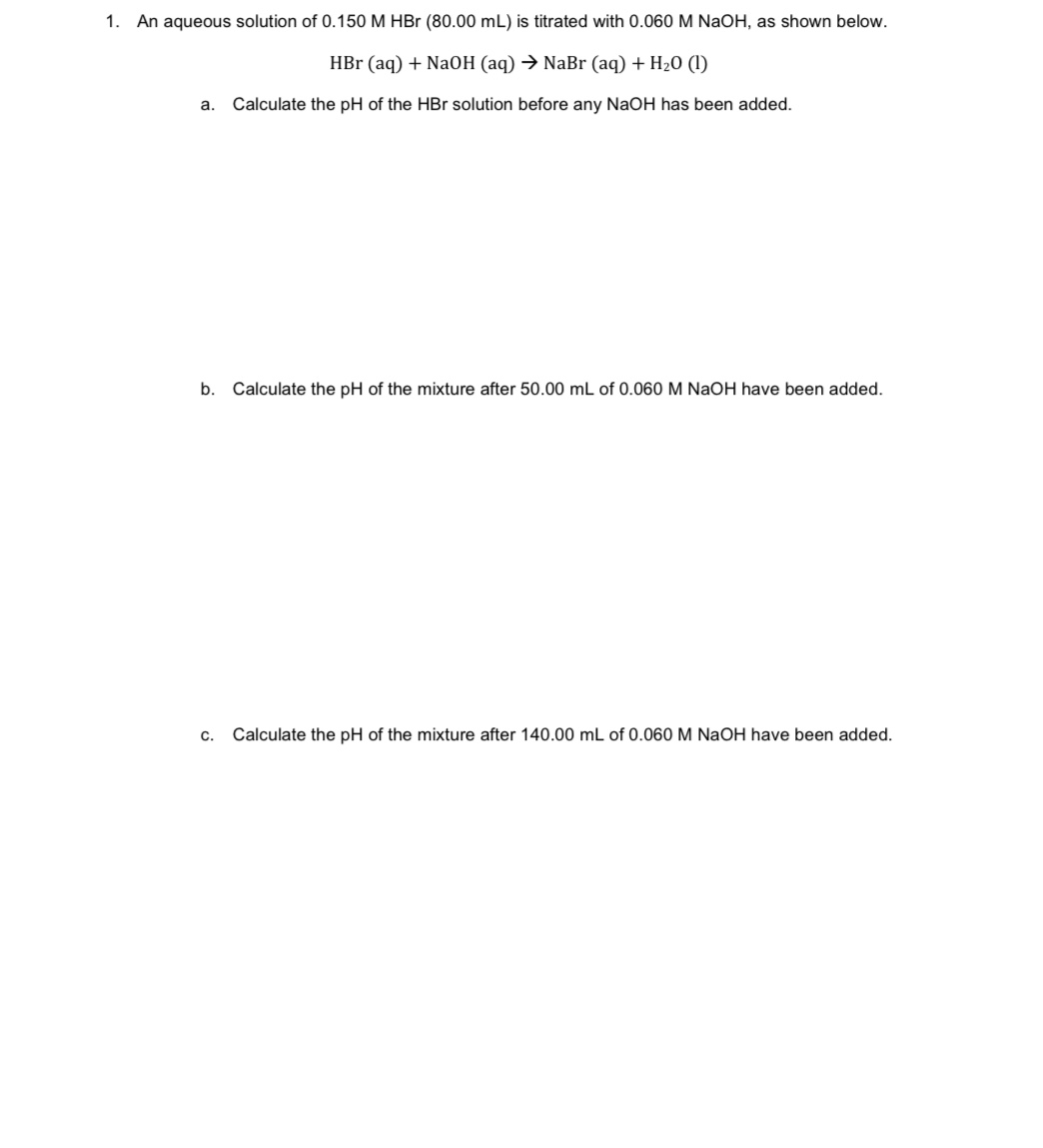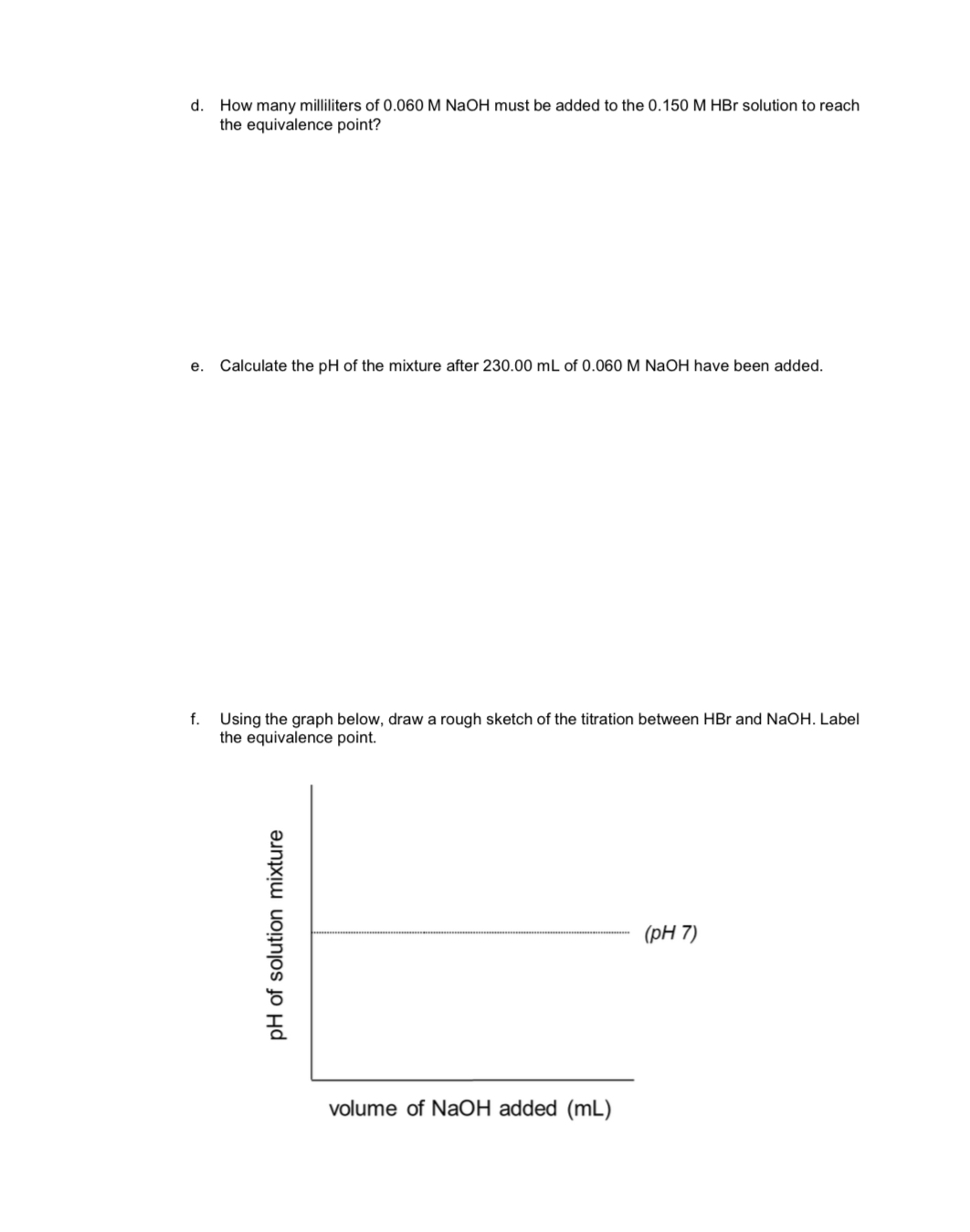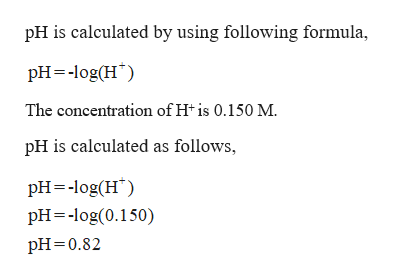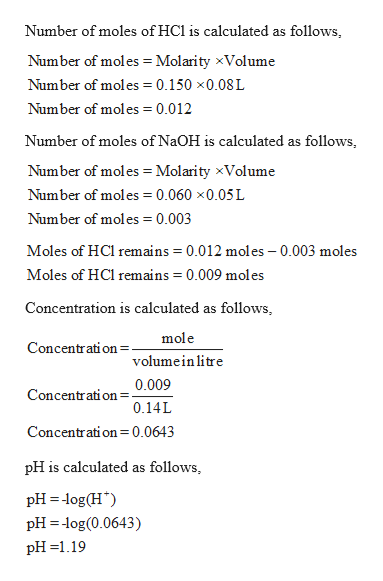# 1.An aqueous solution of 0.150 M HBr (80.00 mL) is titrated with 0.060 M NaOH, as shown below.HBr (aq)NaOH (aq)NaBr (aq) + H20 (1)Calculate the pH of the HBr solution before any NaOH has been addedа.b. Calculate the pH of the mixture after 50.00 mL of 0.060 M NaOH have been added.Calculate the pH of the mixture after 140.00 mL of 0.060 M NaOH have been addedc.

Question
30 viewshelp_outlineImage Transcriptionclose1. An aqueous solution of 0.150 M HBr (80.00 mL) is titrated with 0.060 M NaOH, as shown below. HBr (aq)NaOH (aq)NaBr (aq) + H20 (1) Calculate the pH of the HBr solution before any NaOH has been added а. b. Calculate the pH of the mixture after 50.00 mL of 0.060 M NaOH have been added. Calculate the pH of the mixture after 140.00 mL of 0.060 M NaOH have been added c. fullscreenfullscreen
check_circle

Step 1

Given,

0.150 M HBr is titrated with 0.060 M of NaOH.help_outlineImage TranscriptionclosepH is calculated by using following formula pH log(H The concentration of H is 0.150 M. pH is calculated as follows, pH log(H pH log(0.150) pH 0.82 fullscreen
Step 2

(b)  pH of the mixture has to be calculated after 50 ml of 0.060 M NaOH is added.

Number of moles...help_outlineImage TranscriptioncloseNumber of moles of HCl is calculated as follows, Number of moles = Molarity xVolume Number of moles 0.150 x0.08 L Number of moles 0.012 Number of moles of NaOH is calculated as follows, Number of moles= Molarity xVolume Number of moles 0.060 x0.05 L Number of moles 0.003 Moles of HCl remains 0.012 mol es - 0.003 moles Moles of HCl remains 0.009 moles Concentration is calculated as follows mole Concentration=. volumein litre 0.009 Concentrati on 0.14L Concentration=0.0643 pH is calculated as follows pH log(H pH log(0.0643) pH 1.19 fullscreen

### Want to see the full answer?

See Solution

#### Want to see this answer and more?

Solutions are written by subject experts who are available 24/7. Questions are typically answered within 1 hour.*

See Solution
*Response times may vary by subject and question.
Tagged in

### Chemistry